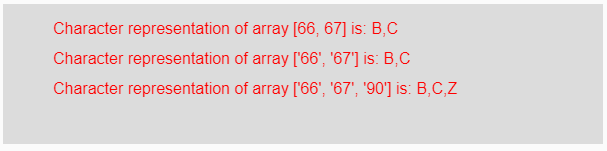# p5.js | char() function

The char() function in p5.js is used to convert the given string or number value into its corresponding single-character string representation. If the input parameter is a string value then at first it gets converted into its integer equivalent internally after that its single-character string is represented as output and when an array of strings or number is taken as the parameter then it gives output as an individual single-character strings for each element of the array.

Syntax:

`char( value )`

Parameters: This function accepts single parameter value which is to be converted into its single-character string representation. This value might be a number, string of numbers and an array of strings or numbers.

Return Value: It returns the converted single-character string representation.

Below programs illustrate the char() function in p5.js:

Example 1: This example uses char() function to convert input value into its corresponding character.

 `function` `setup() {  ` `  `  `    ``// Creating Canvas size ` `    ``createCanvas(600, 230);  ` `}  ` `  `  `function` `draw() {  ` `      `  `    ``// Set the background color  ` `    ``background(220);  ` `    `  `    ``// Initializing some values ` `    ``let Value1 = 66; ` `    ``let Value2 = 67; ` `    ``let Value3 = ``"65"``; ` `    ``let Value4 = 62;  ` `    ``let Value5 = ``"62"``; ` `    ``let Value6 = 90; ` `    `  `    ``// Calling to char() function. ` `    ``let A = char(Value1); ` `    ``let B = char(Value2); ` `    ``let C = char(Value3); ` `    ``let D = char(Value4); ` `    ``let E = char(Value5); ` `    ``let F = char(Value6); ` `      `  `    ``// Set the size of text  ` `    ``textSize(16);  ` `      `  `    ``// Set the text color  ` `    ``fill(color(``'red'``));  ` `    `  `    ``// Getting character representation ` `    ``text(``"Character representation of value 66 is: "` `+ A, 50, 30); ` `    ``text(``"Character representation of value 67 is: "` `+ B, 50, 60); ` `    ``text(``"Character representation of string '65' is: "` `+ C, 50, 90); ` `    ``text(``"Character representation of value 62 is: "` `+ D, 50, 110); ` `    ``text(``"Character representation of string '62' is: "` `+ E, 50, 140); ` `    ``text(``"Character representation of value 90 is: "` `+ F, 50, 170); ` `}  `

Output:Example 2: This example uses char() function to convert input value into its corresponding character.

 `function` `setup() {  ` `  `  `    ``// Creating Canvas size ` `    ``createCanvas(600, 140);  ` `}  ` `  `  `function` `draw() {  ` `      `  `    ``// Set the background color  ` `    ``background(220);  ` `    `  `    ``// Initializing some values ` `    ``let Value1 = [66, 67]; ` `    ``let Value2 = [``"66"``, ``"67"``]; ` `    ``let Value3 = [``"66"``, ``"67"``, ``"90"``] ` `    `  `    ``// Calling to char() function. ` `    ``let A = char(Value1); ` `    ``let B = char(Value2); ` `    ``let C = char(Value3); ` `      `  `    ``// Set the size of text  ` `    ``textSize(16);  ` `      `  `    ``// Set the text color  ` `    ``fill(color(``'red'``));  ` `    `  `    ``// Getting character representation ` `    ``text(``"Character representation of array [66, 67] is: "` `+ A, 50, 30); ` `    ``text(``"Character representation of array ['66', '67'] is: "` `+ B, 50, 60); ` `    ``text(``"Character representation of array ['66', '67', '90'] is: "` `+ C, 50, 90); ` `}      `

Output:Reference: https://p5js.org/reference/#/p5/char

My Personal Notes arrow_drop_upCheck out this Author's contributed articles.

If you like GeeksforGeeks and would like to contribute, you can also write an article using contribute.geeksforgeeks.org or mail your article to contribute@geeksforgeeks.org. See your article appearing on the GeeksforGeeks main page and help other Geeks.

Please Improve this article if you find anything incorrect by clicking on the "Improve Article" button below.

Article Tags :

Be the First to upvote.

Please write to us at contribute@geeksforgeeks.org to report any issue with the above content.